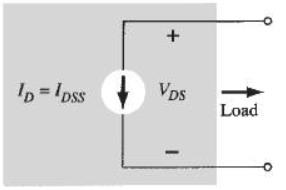Courses

# The PN - Junction, MCQ Test

## 20 Questions MCQ Test GATE Electrical Engineering (EE) 2022 Mock Test Series | The PN - Junction, MCQ Test

Description
This mock test of The PN - Junction, MCQ Test for Electronics and Communication Engineering (ECE) helps you for every Electronics and Communication Engineering (ECE) entrance exam. This contains 20 Multiple Choice Questions for Electronics and Communication Engineering (ECE) The PN - Junction, MCQ Test (mcq) to study with solutions a complete question bank. The solved questions answers in this The PN - Junction, MCQ Test quiz give you a good mix of easy questions and tough questions. Electronics and Communication Engineering (ECE) students definitely take this The PN - Junction, MCQ Test exercise for a better result in the exam. You can find other The PN - Junction, MCQ Test extra questions, long questions & short questions for Electronics and Communication Engineering (ECE) on EduRev as well by searching above.
QUESTION: 1

### What is the effect of cascading the amplifier stages?​

Solution:

Due to cascading voltage gain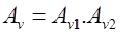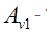Voltage gain of 1st satge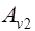- Voltage gain of 2nd stage
So it increases.
While upper cutoff frequency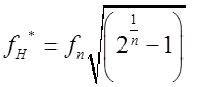While lower cutoff frequency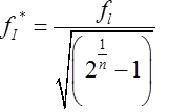Increases compare to single state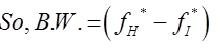As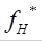decreases and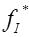increases.

QUESTION: 2

### Generally, the gain of a transistor amplifier falls at high frequencies due to the​

Solution:

The gain of a transistor falls at high frequencies due to the various parasitic capacitances of the devices.

QUESTION: 3

### For the MOSFET shown in the figure, the threshold voltage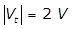and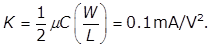The value of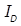(in mA) is ___________.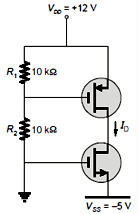Solution: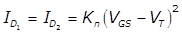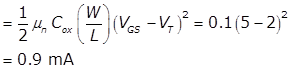QUESTION: 4

The trans conductance gm of the transistor shown in figure is 10 mS. The value of the input resistance RIN   seen by source is -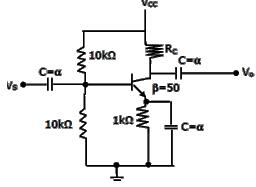Solution:

Input Resistance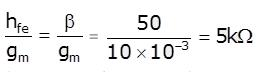But overall input resistance seen from source is
RIN = 10 || 10|| 5
RIN = 2.5 kΩ.

QUESTION: 5

In the circuit shown in figure (a), if the input is given as in figure (b). then the output will be (Assume that the time constant is very large)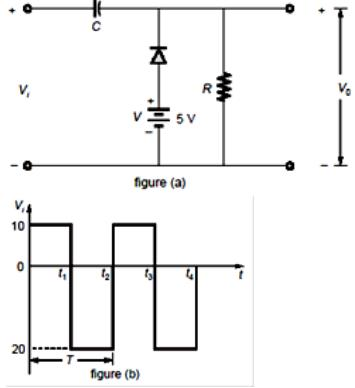Solution:

The analysis will begin with the period t1-t2 of the input signal since the diode is in its short circuit state.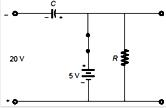The output is across R, but it is also across 5 V battery so V0 = 5V
Applying KVL around the input loop
-20-5 + Vc = 0
Vc= 25 V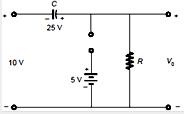the capacitor will therefore charge up to 25 V from t2 to t3 the circuit will appear as shown below by applying KVL in the outside loop,
-10-25+V0 =0
V0 = 35 V
∴ The output waveform will be as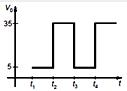QUESTION: 6

The input resistance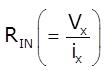of the circuit in figure is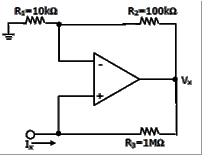Solution:

Here Ix =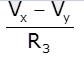But Vy =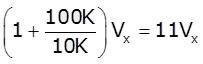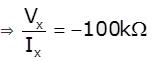Vy = 11 Vx
Ix = (Vx - Vy) / (1M Ω)
= (Vx - 11 Vx) / (106 Ω)
= -10 Vx/ 106
Therefore V/ Ix = 106/ (-10) = -100 K Ω

QUESTION: 7

The following circuit has R = 10kΩ, C = 10μF. The input voltage is a sinusoid at 50Hz with an rms value of 10V. Under ideal conditions, the current is from the source is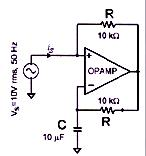Solution: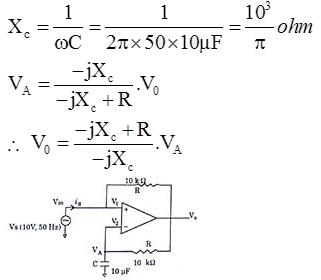Assuming virtual ground,
Vm = VA = 10V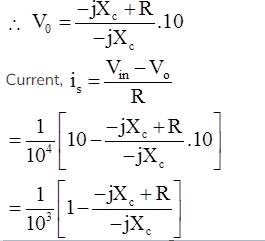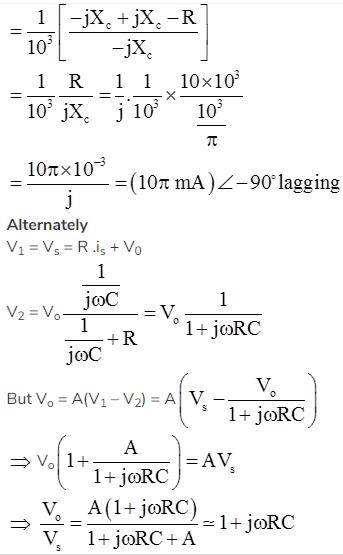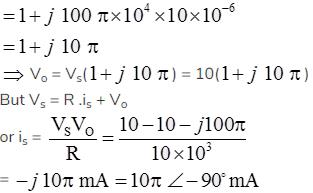QUESTION: 8

For the given n-channel MOSFET, determine the % decrease in the drain current at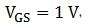if the n-channel between the two n-doped regions is removed. Given,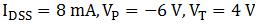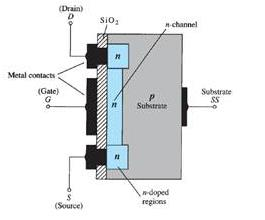Solution:

Given is a depletion-type MOSFET. The drain current is given as,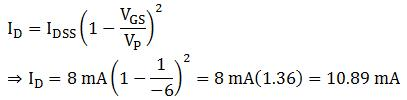When the n-channel between the two-doped regions is removed, it resembles an enhancement-type MOSFET as shown below.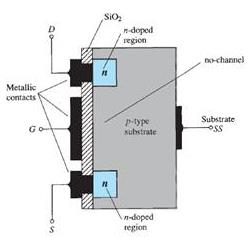For an enhancement-type MOSFET,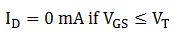Since,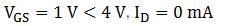Thus, there is a 100% decrease in the drain current.

QUESTION: 9

The feedback used in the circuit shown in figure can be classified as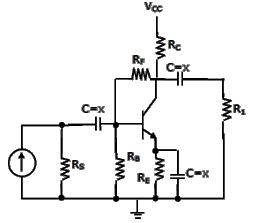Solution:

Equivalent circuit can be drawn with input voltage comparison and current feedback. It is shunt-shunt feedback.

QUESTION: 10

For the given network, determine the output waveform. The circuit components are given below: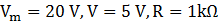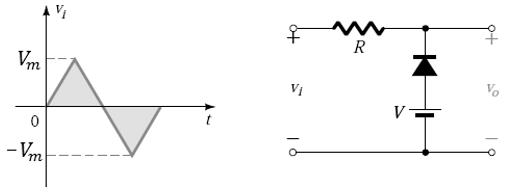Solution:

The output voltage is across the series combination of 5 V supply, and the diode.
In the positive region of the input signal, the diode is an open circuit. The voltage drop across the resistor is zero. Hence,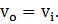There is a change in state at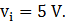Hence, the output is fixed at 5 V until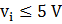, and above that the output waveform is same as the input waveform.
In the negative region of the input signal, the diode is on. When the diode is short-circuited, the output voltage is directly across the 5 V supply, and hence, the output is fixed at 5 V.
Hence, the output waveform is obtained as: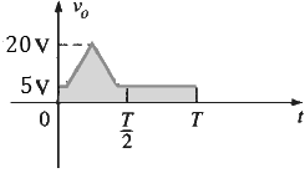QUESTION: 11

In the voltage doubler circuit shown in the figure, the switch ‘S’ is closed at t = 0. Assuming diodes D1 and D2 to be ideal, load resistance to be infinite and initial capacitor voltages to be zero, the steady state voltage across capacitors C1 and C2 will be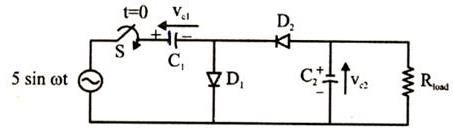Solution:

Since RLOAD is infinite, we can replace with open circuit
C1 will be charged in first +Ve half cycle
VC1 = +5v
C2 will be charged in first –Ve half cycle
VC2 = -10v

QUESTION: 12

A clipper circuit is shown below.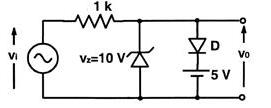Q. which of the following is the transfer characteristics of above circuit?

Solution:

When –0.7 V < Vi < 5.7 V output will following put, because zener diode and normal diodes are off
When Vi < –0.7 V Zener diode forward bias and V0 = –0.7 V
When Vi > 5.7 V Diode is forward bias and Vn = 5.7 V

QUESTION: 13

Including a small resistance in the source (emitter) of a CS (CE) amplifier provides the designer with a tool to improve the performance, that is

Solution:

If there is a small resistance added in the emitter of CE configuration will effect the gain as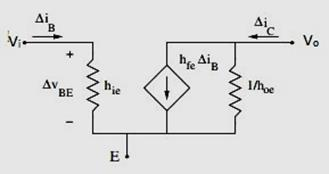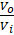=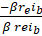=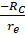=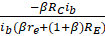In the second case, the gain decreased due to emitter resistance RE. as gain decreases , to maintain gain bandwidth product constant, bandwidth increases.

QUESTION: 14

The transistor in the given circuit should always be in the active region.Take VcE(sat)=0.2V, VEE= 0.7 V The maximum value of Rc in Ω which can be used is __________.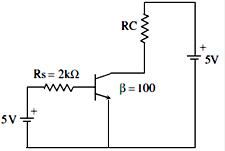Solution: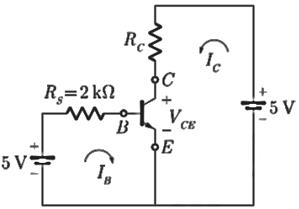Given: VCE(sat) = 0.2
VBE = 0.7
β = 100
Writing KVL around the input loop
5 - IBRS - 0.7 = 0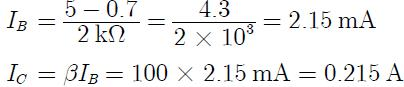Writing KVL around the output loop
5 - ICRC - VCE = 0
VCE = 5 - (0.215)RC
For active region VCE > VCE(sat)
or,      VCE > 0.2 V
So,     5 - 0.215RC > 0.2
0.215RC < 5 - 0.2
Hence RC(min) = 4.8 / (0.215) = 22.32 Ω

QUESTION: 15

In the circuit shown , function of diode D1 is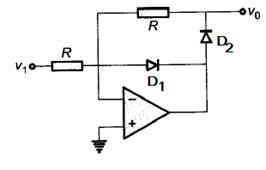Solution:

If D1 would have not been there then for V1<0, D2 ON and op-amp would have been in open loop mode.
In general Op-amp has high open loop gain which may cause the saturation.
By adding D1 saturation is avoided.

*Answer can only contain numeric values
QUESTION: 16

For the network of figure determine the input impedance Zi (in kΩ )Assume Vcc=22V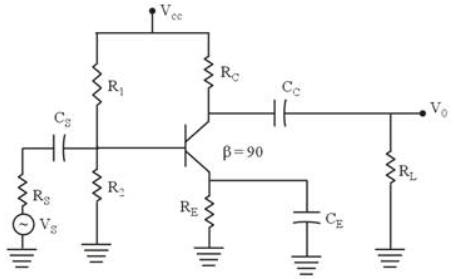RS = 10 kΩ, R1 = 56 kΩ, R2 = 8.2 kΩ
CE = 20 μF, RE = 1.5 kΩ
RC = 6.8 kΩ, CC = 10 μF

Solution: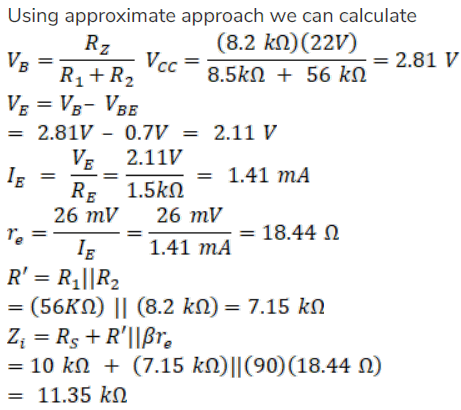*Answer can only contain numeric values
QUESTION: 17

For the common Emitter configuration circuit shown below,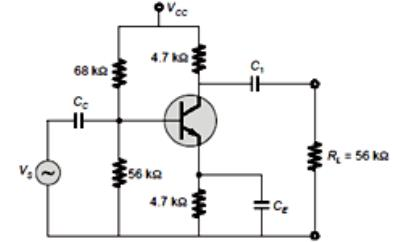If Ic = 1.5mA then the magnitude of voltage gain is __________.

Solution: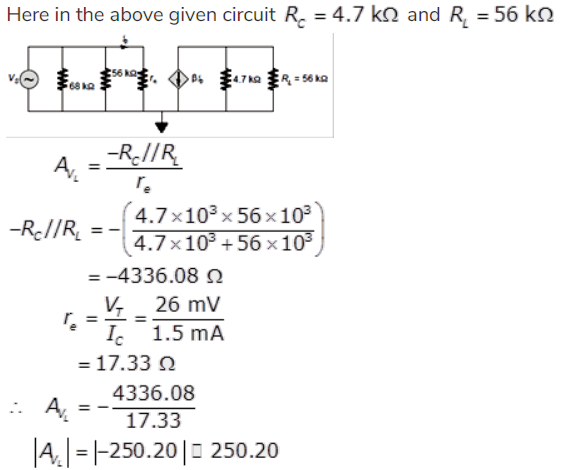QUESTION: 18

For the given fixed-bias configuration, determine the parameters re & ro for its equivalent re  model of the transistor, if the input impedance of the network is 1 kΩ and the output impedance is 4 kΩ.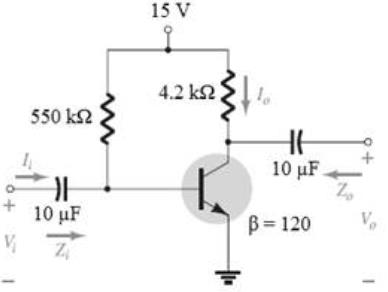Solution:

The ac equivalent circuit for the network is given below.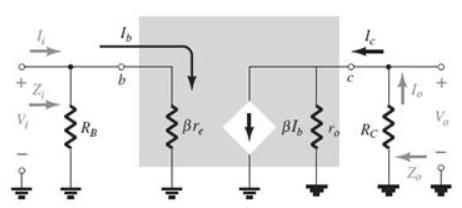The input impedance of the network is given as,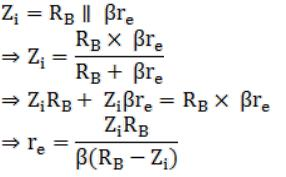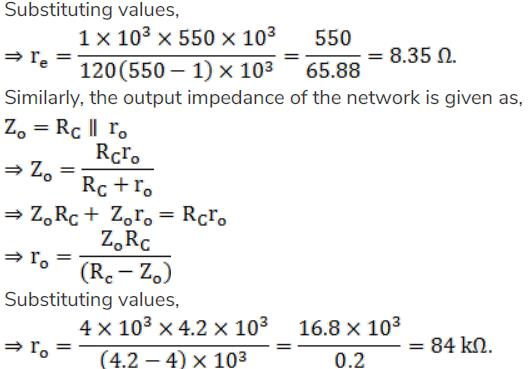QUESTION: 19

Identify the type of connection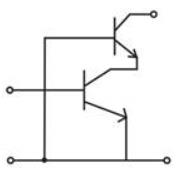Solution:
QUESTION: 20

When the controlling voltage of an n-channel JFET is kept at 0 V, and a voltage greater than the pinch-off voltage is applied between the drain and the source terminal, the JFET:

Solution:

The transfer characteristics for an n-channel JFET when the controlling voltage, VGS = 0V  is shown below.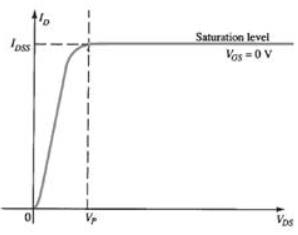It can be seen from the characteristics that when the voltage between the drain and source (VDS) becomes greater than the pinch-off voltage (VP), the drain current remains essentially the same, as the region between the two depletion regions will increase in length. The drain current ID is fixed at the value ID = IDSS, where IDSS is the maximum drain current.
Hence, the JFET acts as a current source as shown below, for VGS = 0V, VDS>Vp